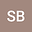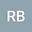A Multiplicative calculus approach to solve applied nonlinear models
••Sonia Bhalla
Chandigarh University

Corresponding Author:[email protected]

Author Profile## Abstract

Problems like population growth, continuous stirred tank reactor (CSTR) and ideal gas are studied from the last four decades in the field medicine science, Engineering and applied science, respectively. One of the main motivation was to understand the pattern of such issues and how to fix them. With the help of applied Mathematics, such problems can be converted or modeled by nonlinear expressions with similar properties and the required solution can be obtained by iterative techniques. In this manuscript, we proposed a new iterative scheme for multiple roots (without prior knowledge of multiplicity m) by adopting multiplicative calculus rather than the standard calculus. The base of our scheme is on the well-known Schröder method and we retain the same second-order of convergence. In addition, we extend the order of convergence from second to fourth by constructing a two-step joint Schröder scheme with hybrid approach of ordinary and multiplicative calculus. Some numerical examples are tested to find the roots of nonlinear equations and results are found to be competent as compared to ordinary derivative methods. Finally, the convergence of schemes is also analyzed by basin of attractions that also support the theoretical aspects.